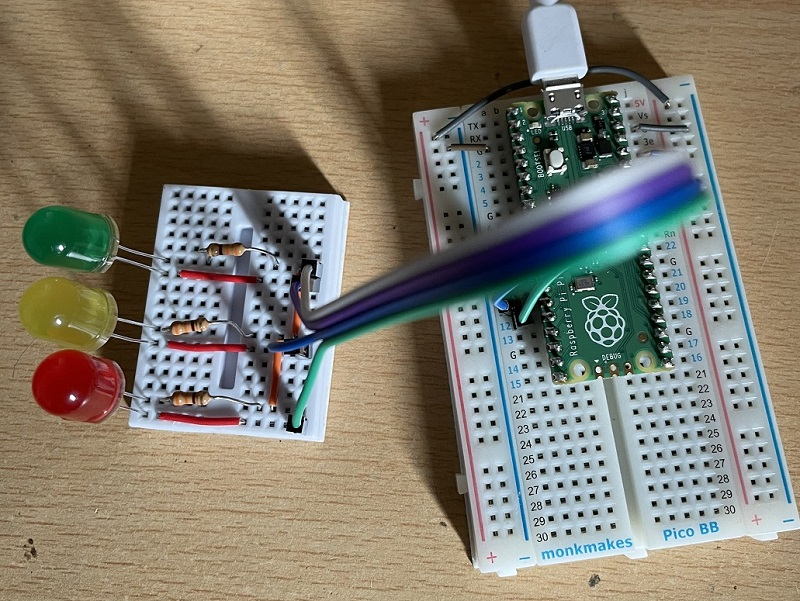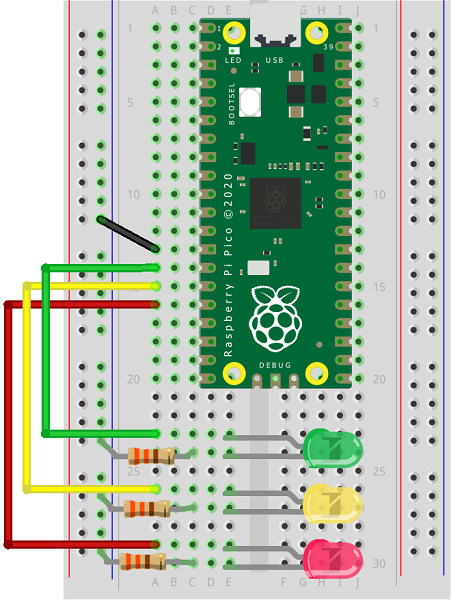# Raspberry Pi Pico Multiple LEDs

I have connected three LEDs in this project. The anodes (positive) pins of the LEDs are connected to pins 10, 11 and 12 on the Pico. The cathodes (negative) pins of the LEDs are connected via a 330 Ohm resistor to GND. In this picture I have used a mini breadboard to make my LED circuit and connected the resistors together and then to ground.This a Fritzing diagram for the same circuit which might be easier to follow than the picture,Using the same principle as we have seen for a single LED we can connect several at a time. We just use different pins. When we have more than one LED, it makes sense to use lists to make some of the tasks easier to carry out.

```from machine import Pin
from time import sleep

pins = [10,11,12]
leds = []

for p in pins:
leds.append(Pin(p, Pin.OUT))

while True:
leds.value(1)
sleep(0.5)
leds.value(0)
leds.value(1)
sleep(0.5)
leds.value(0)
leds.value(1)
sleep(0.5)
leds.value(0)
sleep(0.5)
```

### Binary Counting

If we have a group of LEDs in a row, we can treat them like they are binary place values.

```from machine import Pin
from time import sleep

pins = [10,11,12]
leds = []

for p in pins:
leds.append(Pin(p, Pin.OUT))

def show(n):
bits = [n >> i & 1 for i in range(len(leds)-1,-1,-1)]
for i in range(len(leds)):
leds[i].value(bits[i])

while True:
for i in range(8):
show(i)
sleep(0.5)
sleep(3)
```

The subroutine in this program starts by using a list comprehension to create a list of 1s and 0s corresponding to the bits that are set for the value of n. It then sets the value of the LEDs to those bit values.

In the While loop, the loop counts from 0 to 7. 7 is the largest value that can be represented using 3 place values. If you add an LED, you'd be able to count to 15. Make it a total of 8 LEDs and you can represent numbers up to 255 (a byte).